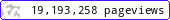•••••MadAsMaths.com :: Project Maths :: Lessons :: Algebra

PROJECT MATHS - LESSONS - ALGEBRA

These presentations contain lessons on a variety of algebraic topics.

Note that this is a licensed product if it is to be used for “classroom teaching” so schools or academic institutions must first purchase a licence. Click HERE to contact me.

 3d_coordinates.pptalgebra_notation_introduction.pptalgebra_with_curved_areas_1.pptalgebra_with_curved_areas_2.pptalgebra_with_curved_areas_3.pptalgebraic_expressions.pptalgebraic_fractions.pptco_ordinates_intro.pptcollecting_terms.pptcomplete_the_square.pptcubic_plotting.pptcurve_intro.pptequation_modelling_6+.pptequation_modelling_and_solving_intro.pptequation_solving_6+.pptequation_solving_by_balancing.pptequation_solving_by_flow_diagrams.pptequation_solving_by_inverse_operations.pptequations_from_text_intro_or_starter_.pptexpanding_brackets.pptexpanding_brackets_harder.pptexpanding_double_brackets.pptexponential_growth_and_decay.pptexponential_plotting.pptfactorisation_by_common_factor_and_identities.pptfactorising_quadratics.pptformula_intro.pptformula_rearrange_intro_by_balancing.pptformulae_rearranging.pptformulae_rearranging_gcse_higher.pptformulae_sub_into_temp_distance_formulae.pptformulae_substitution_intro.pptgradient.pptgradient_formally.pptgraphs_and_equations_gcse_higher.pptgraphs_distance_time.pptgraphs_for_solving_equations_higher_gcse.pptgraphs_from_context.pptgraphs_intro_conversion.pptgraphs_line_graphs.pptgraphs_speed_time.pptidentity_use.pptindicial_equations.pptinequalities.pptinequalities_simultaneous.pptinequality_regions.pptinvestigation_2d_and_3d_sequences_investigation.pptinvestigation_square_pattern_sequences.pptinvestigation_squares_areas_and_sequences.pptinvestigation_squares_on_a_grid_and_algebra.pptinvestigation_the_step_investigation.pptinvestigation_towers_of_hanoi.pptinvestigation_triangle_square_numbers.pptlines_functions_graphs_1.pptlines_functions_graphs_2.pptlines_intro_no_tables.pptlines_parallel_to_axes.pptproofs_intro_or_problem_.pptproportionality_and_graphs.pptproportionality_formally.pptquadratic_equations.pptquadratic_equations_worded.pptquadratic_formula.pptquadratic_plotting.pptquadratic_solution_graphically.pptquadratics_by_completing_the_square.pptreciprocal_plotting.pptrecurrence_relations.pptsequences_algebraic_generation.pptsequences_algebraic_generation_formally.pptsequences_generation_from_a_rule.pptsequences_intro.pptsequences_linear_formally.pptsequences_non_linear_nth_term.pptsequences_nth_term.pptsequences_quadratic.pptsequences_quadratic_nth_term.pptseries_summing.pptsimulataneous_equations_worded.pptsimult_equation_by_substitution.pptsimult_equations_non_linear.pptsimultaneous_equations_elimination.pptsimultaneous_equations_graphically.pptsubstitution.pptsubstitution_negative.ppttangents_and_normals.ppttranformations_of_functions_and_graphs.ppttrigonometric_plotting.ppt